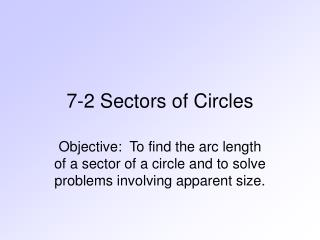# 7-2 Sectors of Circles - PowerPoint PPT PresentationDownload Presentation7-2 Sectors of Circles

7-2 Sectors of CirclesDownload Presentation## 7-2 Sectors of Circles

- - - - - - - - - - - - - - - - - - - - - - - - - - - E N D - - - - - - - - - - - - - - - - - - - - - - - - - - -
##### Presentation Transcript

1. 7-2 Sectors of Circles Objective: To find the arc length of a sector of a circle and to solve problems involving apparent size.

2. Sectors of Circles A sector of a circle, shaded below, it is the region bounded by a central angle and the intercepted arc.

3. Sectors of Circles Example 1: The radius of a pizza is 6 cm. mROQ = 60o. Find the area of the slice OQR. R 6 cm 60° O Q

4. Sectors of Circles Example 2: The radius of a cake is 7 cm. mROP = 130o. Find the area of the slices OPR. R 7 cm O 130° P

5. Arc Length and Area of a Sector of a Circle In general, the following formulas for the arc length s and area K of a sector with central angle  . If  is in degrees, then the arc length and the area of a sector is: If  is in radians, then the arc length and the area of a sector is:

6. r=9 s 30◦ Find the arc length and area of the sector The arc length is: Area:

7. A sector of a circle has perimeter 16 cm and area 15cm². Find its radius r and arc length s. P = 2r + s = 16 s = 16 – 2r When r = 5 cm s = 16 - 2(5) = 6 cm When r = 3 cm s = 16 – 2(3) = 10 cm 1/2rs = 15 rs = 30 r(16 – 2r) = 30 16r – 2r² -30 = 0 r² - 8r + 15 = 0 (r – 5)(r – 3) = 0 r = 5 r = 3

8. A record with diameter 12 in. turns at 33 1/3 rpm. Find the distance that a point on the rim travels in one minute. One revolution is 360˚ or radians. Arc length formula Distance traveled in one minute. S ≈ 1257 in. ≈ 105 ft.

9. Apparent Size When there is nothing in our field of vision against which to judge the size of an object, we perceive the object to be smaller when it is farther away. For example, the sun is much larger than the moon, but we perceive the sun to be about the same size as the moon because the sun is so much farther from Earth. So, how big an object looks depends not only on its size but also on the angle that it subtends at our eyes. The measure of this angle is called the object’s apparent size.

10. Example 2 Jupiter has an apparent size of 0.01o when it is 8 x 108 km from Earth. Find the approximate diameter of Jupiter. As the diagram above indicates, the diameter of Jupiter is approximately the same as the arc length of a sector with central angle 0.01o and radius 8 x 108 km. r = 8 x 108 Diameter≈ s s ≈ 140,000 km

11. ASSIGNMENT • Page 265 1-13